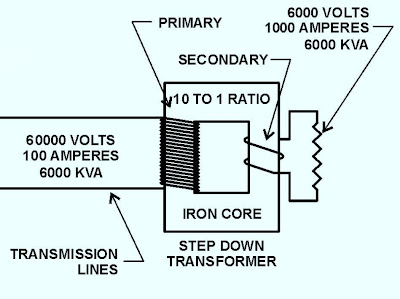### Step-down Power transformers

Step-down Power transformers (Receiving station transformers see fig.1 )fig (1) : Transmission Substation & Receiving station Transformers

Function:
• These transformers take the voltage down from the transmission voltage to an appropriate distribution voltage.

Available ratings:
• The power rating of stop down transformers may range up to power rating of the transmission line. (see fig.2)

fig (2): 40 MVA, 275 KV, 3 PHASE, 50 HZ step-down power transformer

• These transformers have several different distribution voltage levels (see fig.3) As the supply system branches out before the voltage is finally taken down to the final consumption voltage level which may be an industrial plant or other.

fig (3): Different distribution voltage levels

Features:
They are mostly regulating transformers and their turn ratio often controlled by means of voltage regulation relays in order to keep the output voltage constant.

They frequently equipped to vary the turn ratio ±15% in steps 1-1.5% by means of on-load tap changer.

Theory of operation: see fig.4

When an AC supply is connected to the primary coil of a transformer, electrons flow through the coil due to the primary voltage. Alternating current varies, and accompanying magnetic flux varies, cutting both transformer coils and inducing voltage in each coil circuit.

The voltage induced in the primary circuit opposes the applied voltage and is known as back voltage or back electro-motive-force (back EMF).fig (4): theory of operation for step-down transformer

If the small amount of transformer loss is ignored, the back-voltage (back EMF) of the primary must equal the applied voltage. The magnetic field, which induces the back-voltage in the primary, also cuts the secondary coil resulting in an induced voltage in the secondary coil governed by the equation in fig. which state that:

“The total induced voltage in each winding is proportional to the number of turns in that winding”While,

E1 : the primary voltage

I1 : the primary current

E2 : the secondary voltage

I2 : the secondary current

N1 : the primary turns

N2 : the secondary turns

Notes extracted from above equation:

• The current is inversely proportional to both voltage and number of turns. This means (as discussed earlier) that if voltage is stepped up, the current must be stepped down and vice versa. The number of turns remains constant unless there is a tap changer.
• When the number of turns or voltage on the secondary of a transformer is greater than that of the primary, it is known as a step-up transformer And When the number of turns or voltage on the secondary is less than that of the primary, it is known as a step-down transformer.
• Note that kVA remains constant throughout the above circuit on both sides of each transformer, which is why they are called constant wattage devices.
• Efficiencies of well-designed power transformers are very high, averaging over 98 percent (%). The only losses are due to core losses, maintaining the alternating magnetic field, resistance losses in the coils, and power used for cooling. The main reason for high efficiencies of power transformers, compared to other equipment, is the absence of moving parts. Transformers are called static AC machines.

In the next topic, I will show other type of power transformers which is System Intertie transformer.